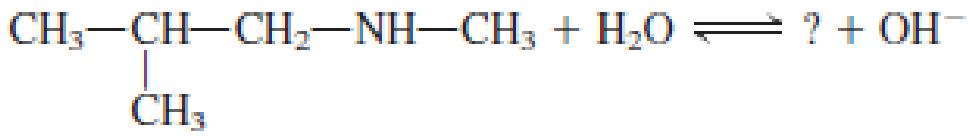Chapter 6, Problem 6.42EP### Organic And Biological Chemistry

7th Edition
STOKER + 1 other
ISBN: 9781305081079

#### Solutions

Chapter
Section### Organic And Biological Chemistry

7th Edition
STOKER + 1 other
ISBN: 9781305081079
Textbook Problem

# Show the structures of the missing substance(s) in each of the following acid-base equilibria.a. Trimethylamine + H2O ⇌ ? + ?

(a)

Interpretation Introduction

Interpretation:

In the given acid‑base equilibria, the structure of missing substance has to be shown.

Concept Introduction:

Amines are a class of organic compounds.  They are derivatives of ammonia.  Similar to the nitrogen atom in ammonia, the amine nitrogen also has a lone pair of electrons on it.  This means that amines can act as proton acceptors.  When an amine is added to water a proton is transferred to the nitrogen atom.  The resulting solution is a basic solution.  This contains ammonium ions and hydroxide ions.

Substituted ammonium ion is the one in which one or more alkyl, aryl, or cycloalkyl groups are substituted instead of hydrogen atoms.  There are two important generalizations that is applied to the substituted ammonium ion and they are,

• Substituted ammonium ions are not neutral molecules.  They are charged species.
• Nitrogen atom has four bonds in ammonium ion or substituted ammonium ion.  The species is positively charged as the fourth bond formed is a coordinate covalent bond.
Explanation

Given acid‑base equilibria reaction is,

In the above reaction acid is water and base is the amine.  Water acts as proton donor and amine acts as proton acceptor to form ammonium ion and hydroxide ion...

(b)

Interpretation Introduction

Interpretation:

In the given acid‑base equilibria, the structure of missing substance has to be shown.

Concept Introduction:

Amines are a class of organic compounds.  They are derivatives of ammonia.  Similar to the nitrogen atom in ammonia, the amine nitrogen also has a lone pair of electrons on it.  This means that amines can act as proton acceptors.  When an amine is added to water a proton is transferred to the nitrogen atom.  The resulting solution is a basic solution.  This contains ammonium ions and hydroxide ions.

Substituted ammonium ion is the one in which one or more alkyl, aryl, or cycloalkyl groups are substituted instead of hydrogen atoms.  There are two important generalizations that is applied to the substituted ammonium ion and they are,

• Substituted ammonium ions are not neutral molecules.  They are charged species.
• Nitrogen atom has four bonds in ammonium ion or substituted ammonium ion.  The species is positively charged as the fourth bond formed is a coordinate covalent bond.

(c)

Interpretation Introduction

Interpretation:

In the given acid‑base equilibria, the structure of missing substance has to be shown.

Concept Introduction:

Amines are a class of organic compounds.  They are derivatives of ammonia.  Similar to the nitrogen atom in ammonia, the amine nitrogen also has a lone pair of electrons on it.  This means that amines can act as proton acceptors.  When an amine is added to water a proton is transferred to the nitrogen atom.  The resulting solution is a basic solution.  This contains ammonium ions and hydroxide ions.

Substituted ammonium ion is the one in which one or more alkyl, aryl, or cycloalkyl groups are substituted instead of hydrogen atoms.  There are two important generalizations that is applied to the substituted ammonium ion and they are,

• Substituted ammonium ions are not neutral molecules.  They are charged species.
• Nitrogen atom has four bonds in ammonium ion or substituted ammonium ion.  The species is positively charged as the fourth bond formed is a coordinate covalent bond.

(d)

Interpretation Introduction

Interpretation:

In the given acid‑base equilibria, the structure of missing substance has to be shown.

Concept Introduction:

Amines are a class of organic compounds.  They are derivatives of ammonia.  Similar to the nitrogen atom in ammonia, the amine nitrogen also has a lone pair of electrons on it.  This means that amines can act as proton acceptors.  When an amine is added to water a proton is transferred to the nitrogen atom.  The resulting solution is a basic solution.  This contains ammonium ions and hydroxide ions.

Substituted ammonium ion is the one in which one or more alkyl, aryl, or cycloalkyl groups are substituted instead of hydrogen atoms.  There are two important generalizations that is applied to the substituted ammonium ion and they are,

• Substituted ammonium ions are not neutral molecules.  They are charged species.
• Nitrogen atom has four bonds in ammonium ion or substituted ammonium ion.  The species is positively charged as the fourth bond formed is a coordinate covalent bond.

### Still sussing out bartleby?

Check out a sample textbook solution.

See a sample solution

#### The Solution to Your Study Problems

Bartleby provides explanations to thousands of textbook problems written by our experts, many with advanced degrees!

Get Started

#### Find more solutions based on key concepts